# Determining and Understanding the Yield Stress of Complex Fluids

Flow of a number of complex fluids like surfactant mesophases, emulsions and network forming polymers is induced only when the applied stress is more than a specific value, which is called the yield stress. Such behavior is termed as yield flow behavior.

When subjected to a force below the yield stress, the sample will undergo elastic deformation, whereas the sample will flow like a liquid when subjected to forces above the yield stress. The structural frame of fluids exhibiting a yield stress extends throughout the volume of the system. The structure of the dispersed phase and corresponding interactions govern the strength of this skeleton.

Generally, the viscosity of the continuous phase is low, but when high volume fractions are present in a dispersed phase and/or in the presence of strong interactions, the viscosity increases drastically, exhibiting near solid like behavior.

Solid-like complex fluids undergo strain hardening when subjected to shearing at low shear rates and below their critical strain. The solid-like behavior of fluids is caused by the stretching of the elastic elements in the shear field. As the elastic elements near their critical strain, the structure starts breaking down resulting in shear thinning (strain softening) and subsequent flow.

Yield stress is the stress at which the breakdown of the structural frame occurs, and the related strain is the yield strain. Figure 1 represents the mechanical analogues depicting the process with the help of a spring in parallel with a damper for a viscoelastic solid; and with a spring and a dashpot in case of a gel.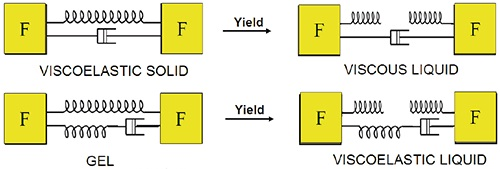Figure 1. Illustration showing mechanical analogues and associated yielding for a viscoelastic solid and a gel.

For both scenarios, the spring prevents the plastic deformation of the material, meaning it needs to be broken first. For visoelastic solids the yielded material behaves as a Newtonian liquid. For the gel, the yielding transforms it into a viscoelastic liquid that exhibits thinning behavior. More complex analogues of spring and dashpot combinations are required for the description of real materials.

When observing a real microstructure under the microscope the components that cause this behavior are visible. Close or ordered packing of the dispersed phase in emulsions and foams is responsible for the solid-like behavior. In the case of polymer gels this behavior is caused by molecular interactions or associations.

Although there are a number of real life examples of yield stress, such as squeezing of toothpaste, the concepts behind true yield stress are debatable. Rapid deformation of an entangled polymer systems or a glassy liquid causes solid-like behavior, while longer deformation times cause liquid-like behavior meaning such materials do not possess a true yield stress.

On sufficiently long timescales, all materials exhibit creep or flow, and as a consequence a number of materials considered to have a true yield stress are in reality highly viscous liquids. Due to the subjective behavior, the term apparent yield stress is generally used, and it is considered to represent the critical stress at which there is a clear drop in viscosity (Figure 2).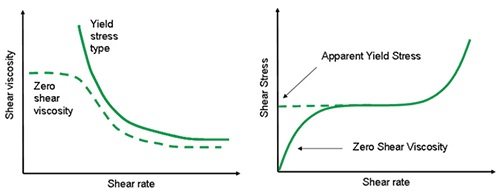Figure 2. Illustration showing an expected flow curve for a material with a true yield stress and a zero shear viscosity (left) and a material which appears to have a yield stress but shows viscous behavior at much lower shear rates. (right)

The differentiation is effectively shown at low shear rates because the material may seem to have a yield stress within a limited range of shear rate, but at very low shear rates, a zero shear viscosity plateau can be seen. However, measurement artifacts like wall slip, instrument resolution and thixotropy need to be considered when such distinctions are made.

The true yield stress is a significant factor for short time processes like stirring and extrusion, pumping, and to an extent for long-term processes influenced by gravity like sedimentation.

Determining the yield stress as a true material constant is challenging because the value relies on the measurement technique used. Yield stress is determined by combining a number of approaches rather than a single method. Time is one of the factors that affects the measured value of yield stress.

Due to the thixotropic nature of many complex fluids, their structure changes with the duration of the applied shear and/or take infinite time for recovering after yielding. This behavior is significant when samples are loaded before measurement, since the material should yield first. The frequency of testing or the timescale is equally important because viscoelastic materials show different responses based on the rate of deformation and the relaxation behavior of the material.

A rotational rheometer with an inverse frequency correlation with time can be used for examining the micro-structural relaxation processes. Figure 3 shows the observed frequency spectrums and corresponding mechanical analogues.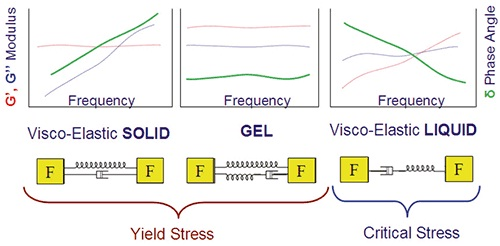Figure 3. Illustration showing some typical frequency profiles for materials with a yield stress/ critical stress and their mechanical analogues.

When G', the modulus pertaining to elasticity, exceeds G", the viscous modulus related to flow, the material is said to have an associated structure, and therefore, a yield stress. In order that the material has a true yield stress, the value of G' must be more than G" at infinitely low frequencies. This is predominant in viscoelastic solids and ideal gels.

In case of viscoelastic liquids, these materials only appear to yield in the frequency range where G' is more than G", thus, considered to exhibit apparent yield or critical stress. Although real materials exhibit all these types of behavior over a broad frequency range, time and measurement constraints limit observations to a limited frequency range.

Temperature is another critical factor: materials are at higher thermal energy states at higher temperatures, requiring only low stress inputs to initiate the flow. As a result, in the absence of thermally induced structural improvements and at high temperatures, yield stress decreases with an increase in temperature.

Measurement of yield stress can be done using different methods, from precise rheometric techniques to primitive, non-specific methods. Slump testing is a non-specific method in which the extent of slumping of a material in the form of a cylindrical volume on a horizontal plane is measured. Another non-absolute method is the Inclined Plane Test in which the equilibrium sample thickness is measured on an inclined surface after a period of flow at the start.

This article will focus on the methods that can be used along with a rotational rheometer, such as stress ramp, creep, stress growth, model fitting and oscillatory techniques.

A comprehensive description of each method along with discussions on the best practices of measurements is also provided. Various techniques for eliminating measurement artifacts such as wall slips, are also provided.

## Model Fitting

Yield stress measurements can be made on a rotational rheometer or viscometer by fitting different models to the measured rheograms and extending to zero shear rate. The Bingham model is the simplest of all models and is frequently used to outline the behavior of concentrated solid particle suspensions in Newtonian liquids.

Such materials exhibit an apparent yield stress, which is followed by a nearly Newtonian flow beyond the yield stress. The mathematical representation of the Bingham model is as below: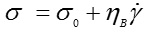In the equation, yield stress is represented by σ0 and Bingham viscosity or plastic viscosity is represented by ηB. The value of Bingham viscosity provides details about the slope of the Newtonian section of the curve, and not the real viscosity value.

The Casson model is an alternative to the Bingham model. In this model, all the components preset in the Bingham equation are raised to the power of 0.5, and as a result, the transition from yield to Newtonian regions is gradual.

The suitability of this model is better than the Bingham model, and it is also a preferred model for the characterization of chocolate and inks. The Casson equation is written below: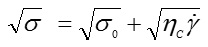In the above equation, σ0 is the value of yield stress and ηC is the value of Casson viscosity that is related to a high shear rate viscosity. Herschel-Bulkley model is another model used in yield stress calculations to describe the non-Newtonian behavior after yielding. This model is fundamentally a power law model, which includes a yield stress term. The equation for this model is as below: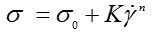In the above equation, K represents the consistency and n represents the shear thinning index, which is a measure of the extent of shear thinning (n <1) or shear thickening (n>1) of the material.

Figure 4 shows the stress-shear rate curves for the Herschel-Bulkley and Bingham type fluid. This representation follows the linear scaling, which differs from the usual logarithmic representation, resulting in different profiles.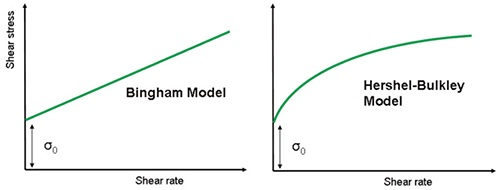Figure 4. Illustration of Bingham and Herschel-Bulkley model fits using linear scaling.

The measurement of the steady shear stress over a range of shear rates helps to choose an appropriate model that fits the data. In this case, the correlation coefficient indicates the suitability of the model. The values of yield stress that are determined by fitting different models is considered as dynamic yield stress and not static yield stress, which is determined by other methods like stress growth and stress ramps.

The minimum stress that is required to maintain flow is the dynamic yield stress, while the stress required for initiating the flow is the static stress. The value of the static stress is higher than dynamic stress in most cases. At the flow initiation stage, it is better to measure the static stresswhile during the flow maintenance or stopping stage it is more appropriate to measure the dynamic stress value.

Apart from those discussed above, there are other models for estimating the value of yield stress, or more specifically the value of critical stress for materials that have a zero shear viscosity. These models are nothing but modified versions of the Ellis and Cross models that are used for viscosity versus shear stress and viscosity versus shear rate data, respectively. The equation for the Ellis model is as below: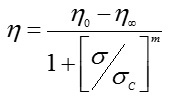In the equation, η represents the viscosity and η0 the zero shear viscosity; and σ is the applied stress and σC is the critical shear stress.

At the critical shear stress, non-linearity sets in. The value of critical shear stress is the asymptotic value of shear stress at infinite viscosity, under the assumption of power law behavior (Figure 5). The degree of non-linearity is given by the shear-thinning index, which is represented as the exponent m. The infinite shear viscosity is η, which is a limiting value of viscosity at extremely high shear rates.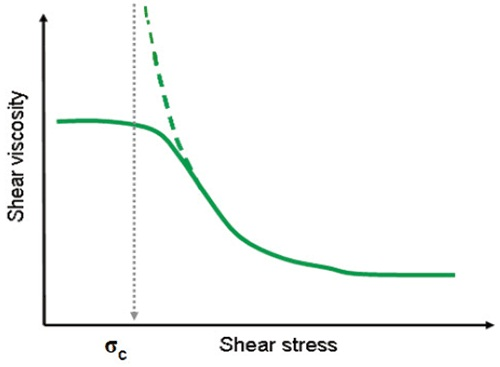Figure 5. Illustration showing an Ellis model fitted to the flow curve of a shear thinning liquid.

## Stress Ramp

The shear stress ramp is one of the simplest and fastest ways of measuring yield stress using a stress-controlled rheometer (Figure 6).

In this method, the value of yield stress is the stress at which a peak in viscosity is observed. The material undergoes elastic deformation before the viscosity peak is attained; therefore, the strain rate is nearly constant despite the linear increase of stress. At the viscosity peak, the elastic structure breaks down or yields, resulting in the flow of the material. At the same time, the shear rate increases steeply, resulting in a reduction in viscosity.

The yield stress may depend on time, in such instances the stress ramp rate becomes more important. For cases like this a constant or a standard value is needed while comparing different samples.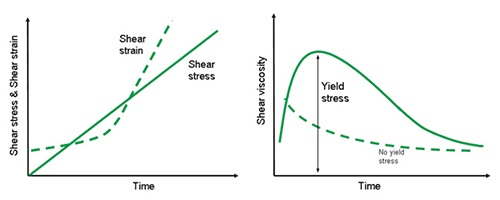Figure 6. Shear stress-strain curve (left) and corresponding viscosity-stress curve (right) for materials with and without a yield stress.

## Stress Growth

Unlike the stress ramp test where a steadily increasing stress is applied and the resulting shear or strain rate is monitored, in the stress growth test, a constantly increasing strain is applied and the stress built over time is monitored. When the strain is less than the critical strain, the material undergoes work hardening due to the stretching of the elastic elements in the shear field.

The structure tends to break down when these elastic elements tends towards critical strain, resulting in shear thinning or strain softening. As a result, the material eventually flows. The shear stress also reaches a peak value simultaneously; at this peak, the stress value is equal to the yield stress. The stress value then levels off at the equilibrium value (Figure 7).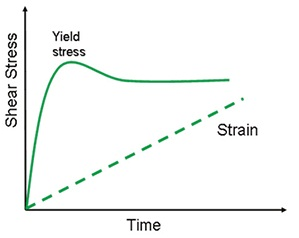Figure 7. Illustration showing the stress evolution of a yield stress material at constant shear rate.

Normally, a low value of shear rate is maintained for these tests to enable time relaxation properties of the material. However, the value of shear rates depends on the application, for example, processes like dispensing occur at short timescales and as a result are associated with higher shear rates.

Whereas, stability to creaming or sedimentation occurs over longer timescales so it needs to be examined at lower shear rates. Due to the dependency of yield stress on time, the measured values are likely to be different. The commonly agreed shear rate is between 0.01 and 1 seconds since the values obtained conform to those obtained from other yield methods.

## Oscillation Amplitude Sweep

The oscillatory stress or strain is increased in this method, and the resulting changes in the elastic modulus (G') or the elastic stress (σ') due to increasing amplitude are measured.

Figure 8 shows the different ways of deriving the yield stress based on the amplitude sweep. In some methods, the initial drop in G' is considered as a measure of the yield point because at this point non-linearity sets in and the structural breakdown occurs. In some other methods, the cross-over of G'/ G" is taken as the yield point, as this indicates the transition from solid to liquid characteristics. The yield zone is the span between these two events because it represents solid to liquid transition.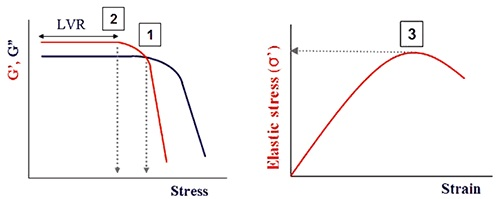Figure 8. Illustration showing points commonly used to determine the yield stress and strain from an oscillation amplitude sweep.

A recent improvisation in the oscillation testing method is the determination of elastic stress component (σ'), which is a function of the strain amplitude and is related to the elastic modulus (G'). The peak value of the elastic stress is considered as the yield stress, and the value of strain corresponding to this stress is taken as the yield strain. Somewhere in between the G'/G" crossover and the initial drop in G' lies the stress value. The value of yield stress obtained by this method is highly reliable and agrees with those obtained from other methods.

A notable point is the dependency of the measured yield stress on the test frequency, based on the relaxation behavior of the material under study. A decrease in the frequency results in a decrease in the G' value for complex fluids, and the yield stress follows suit. The properties of the material at rest are represented more precisely at lower frequencies; hence, the time of test is extended when amplitude sweep is performed at such low frequencies.

As a result, frequency values between 0.01 and 10 Hz are used based on the specific application. Additional care needs to be exercised when a serrated parallel plate is used in the experiment because its geometry oscillates around a fixed position, which results in voids in the material.

## Multiple Creep

The multiple creep method is by far the most accurate ways of determining yield stress. In this method, a row of creep tests are performed by varying the applied stresses and examining the resulting changes in the slope of the compliance vs time curve. The response depends on the characteristic of the tested material (Figure 9).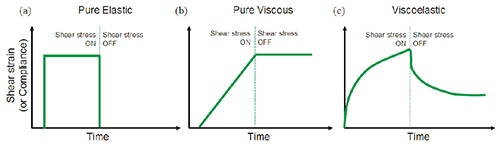Figure 9. Illustration showing the strain response to an applied stress for (a) purely elastic material (b) purely viscous material (c) viscoelastic material.

In this method, emphasis is on the compliance and not the strain, because the applied stress influences the actual strain. Using the following equation, the creep shear compliance (J) can be obtained from the preset shear stress (σ) and the deformation (γ).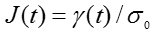The creep curves that are generated at varying stresses can be compared directly using the above equation. As long as the applied stress lies in the linear viscoelastic region and below the critical strain and stress, the J(t) curves overlap each other irrespective of the applied stress.

When these conditions are not met, the material is said to have yielded. It can be seen from Figure 10 that the yield stress of the test material varies between 3 and 4 Pa, because at 4 Pa the curve does not overlay the lower stress data. The test needs to be repeated by increasing the stress in small increments within this range for attaining a more accurate estimate of the yield stress.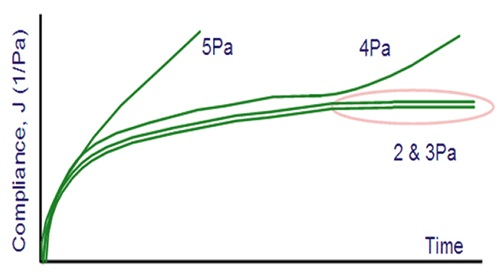Figure 10. Illustration showing a multiple creep test with yielding at 4 Pa.

It is evident from Figure 10 that the test time is an important factor for this method. The yielding begins after a stipulated time at 4 Pa but instantly at 5 Pa. This behavior is due to the structurally dynamic nature of some materials.

The typical test time ranges from 2 to 10 minutes, but in case of materials that relax over as longer period, longer test times are required. The material needs to be relaxed in between creep tests, in order to avoid the accumulation of stresses that would result in artificially low yield stress values. The introduction of a relaxation step between creep tests, where zero stress is applied for a time equivalent to the creep time is essential for avoiding the accumulation of stresses.

## Tangent Analysis

Figure 11 shows the tangent analysis method for determining yield stress.

This method can be used alongside the steady shear and oscillatory techniques. When a single tangent is applied in the linear region of the oscillatory test curve, the value of the yield stress is derived at the point where the curve deviates from this tangent. This represents the end of the linear region shown in Figure 8.

Another way of deriving the yield stress is applying tangents to the linear viscoelastic region and the flow region, and recording intersect of these tangents as the yield stress value. Unlike the modulus-strain data, in tangent analysis the tangents are fitted to stress-strain data. Nevertheless, these two values would be equivalent.

As shown in Figure 11(a), the same method can be followed for a shear stress ramp as well. It is important to plot the data logarithmically when fitting tangents for determining yield stress, as a majority of yielding materials exhibit power law behavior.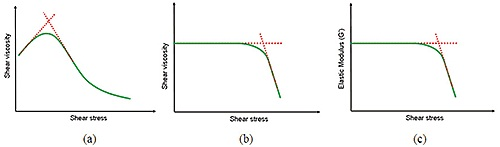Figure 11. Illustration showing yield stress/critical stress determination by tangent analysis using steady shear testing (a and b) and oscillation testing. (c)

## Wall Slip

The measurement system has a bearing on the rheological measurements, especially in the measurement of yield stress. For example, when shear rheological measurements are being made on structured liquids like specific suspensions, foams or emulsions there is a possibility that the measurements are affected by “wall slip”.

The depletion of the dispersed phase that occurs locally near the geometry wall causes wall slip, forming a lubrication layer at the surface. As a result, accurate measurement of bulk rheological properties is not possible, which results in an underestimation of yield stress and true viscosity.

In case of measurements in stiff solid like materials, the friction between the sample and the wall is not sufficient for supporting the applied stress. There are a number of methods available for countering the effect of wall slip, among which the use of serrated or roughened geometries is the most effective method.

Here, the geometry motion is incorporated into the bulk of the liquid, maximizing sample-sample contact by compromising on sample-wall interactions. The roughness of the geometry is chosen based on the size of any dispersed matter and the stiffness of the material, with larger particles and stiffer materials requiring serrated plates. The result of a wall slip that occurs in a concentrated particle suspension when measured using two smooth parallel plates is shown in Figure 12.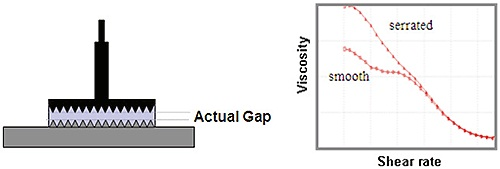Figure 12. Illustration of serrated parallel plates used to minimize slip and associated data for a the flow curve of a dispersion measured with smooth and serrated plates.

The kink or the ‘dog leg’ depicted in the flow curve is usually caused by wall slip, which can be avoided using serrated plates.

## Cone and Plate Measuring Systems

A cone measuring system is most suitable when the plate set up is used on the rheometer because it ensures uniform shear stress across the entire cone surface, which, in turn, ensures uniform yield of the material across the sample radius. The measured value of stress depends on the applied shear rate, which varies with the radius, in the case of parallel plates. Therefore, the yielding of the sample that is exposed to the outer radius of the plate happens before it does in the central region.

In order to avoid the effect of slip, the cones are roughened. For samples that contain large suspensions and/or there is a need for a serrated system due to excessive slippage, then the only way of deriving accurate measurements is using a parallel plate set up.

In these tests, the gap is an important factor because slip effects are predominant for small gaps. This is due to the combined effect of the geometry velocity and the slip velocity at the plate surface. As the gap becomes narrower, the geometry velocity reduces relative to the constant slip velocity. When paste like materials are used, there may be some restrictions on the working gap, which can be used for the precise quantification of yield stress.

This is due to the inhomogeneous flow of material on the application of large gaps, causing fractures across the gap or partial yielding. This yielding is visually evident, and two separate layers that move at different speeds can be seen upon closer observation. A distinct inflection on the shear stress-shear rate curve is also seen.

## Cylinder and Vane Measuring Systems

Vanes and splined geometries are used on a cylinder set up on a rheometer. This arrangement reduces the slip in the same way as a serrated plate system. The vane geometry is preferred for concentrated emulsions and dispersions where slippage is common, since it maximizes the sample-sample contact. The vane tool can be conveniently incorporated into the sample, without causing much disturbance to the structure.

This is of great significance for complex, thixotropic fluids that tend to recover their structure in a finite time after loading, or not at all in some cases. Another advantage of the vane tool is that it can be used along with the product even when it is present in its original container, eliminating the need for transferring the sample to a measurement cup and any structural damage during the transfer.

While making yield stress measurements using the cup and bob system or the vane tool, measurements must be made at the bob wall or the vane edge, instead of the midpoint, as laid down by the ISO3219, DIN53019 standards for viscosity measurement. This measurement procedure is followed because the yielding will initially occur at the bob edge since the stress decreases proportionally with the radial distance from the bob. The rotating vane of the vane tool will draw a circular path in the sample, and hence, can be considered to act like a cylindrical bob made of the sample (Figure 13).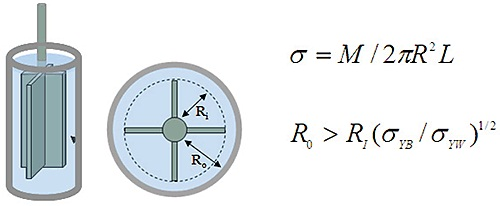Figure 13. Illustration of vane tool in a smooth cup and associated stress equation. M is the torque and L the vane length; σYB is bulk yield stress and σYW the wall yield stress.

Due to the sample-sample contact, there is minimal slip at the periphery of the vane, but in case of improper profiling of the cup surface, the sample at the outer wall surface tends to slip or yield before the sample yields. As the radius from the bob/bane surface increases, the stress decreases. In order to eliminate these effects, a larger measuring gap should be chosen, alternately, a splined or serrated cup or basket may be used.

## Conclusion

Characterization of various complex fluids can be done effectively based on their yield stress values. It is an important factor for understanding a number of real-life processes and applications that use such materials. Evaluating the test type and measurement protocol followed in the test is critical for obtaining relevant, reproducible and robust yield stress data of specific materials.This information has been sourced, reviewed and adapted from materials provided by Malvern Panalytical.

## Citations

• APA

Malvern Panalytical. (2019, September 03). Determining and Understanding the Yield Stress of Complex Fluids. AZoM. Retrieved on October 20, 2020 from https://www.azom.com/article.aspx?ArticleID=12448.

• MLA

Malvern Panalytical. "Determining and Understanding the Yield Stress of Complex Fluids". AZoM. 20 October 2020. <https://www.azom.com/article.aspx?ArticleID=12448>.

• Chicago

Malvern Panalytical. "Determining and Understanding the Yield Stress of Complex Fluids". AZoM. https://www.azom.com/article.aspx?ArticleID=12448. (accessed October 20, 2020).

• Harvard

Malvern Panalytical. 2019. Determining and Understanding the Yield Stress of Complex Fluids. AZoM, viewed 20 October 2020, https://www.azom.com/article.aspx?ArticleID=12448.